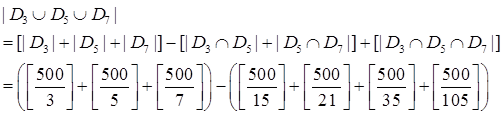# GATE Solved Paper 2017-19 - GATE 2017 Shift 1

>>>>>>>>GATE 2017 Shift 1

• A

271• B

337• C

267• D

270• Option : A
• Explanation :
{integers between 1 to 500 divisible by 3}
{integers between 1 to 500 divisible by 5}
{integers between 1 to 500 divisible by 7}
To find number of integers between 1 to 500 that are divisible by 3 or 5 or 7 is to find(166+100+71) - (33+23+14)+4
337-70+4 = 271

 Inst. No. Instruction i: add R2, R3, R4 i + 1: sub R5, R6, R7 i + 2: cmp R1, R9, R10 i + 3 beq R1, offset
If the target of the branch instruction is i, then the decimal value of the Offset is _____.

Note – Numerical Type question

• A

-15• B

-16• C

-18• D

-12• Option : B
• Explanation :  I1 0-3 2 4-7 I3 8-11 I4 12-15 16-
I4 is the branch instruction & is the target.
0 = 16+ relative value
∴ relative value = -16

• A

13• B

16• C

11• D

17• Option : C
• Explanation :  Given Data As per RSA Algorithm P = 13 Step1: Calculate n = pxq = 13x 17 = 221 Q = 17 Step2: Calculate ϕ(n) = (p-1)(q-1) = (12)(16) = 192 E = 35 Step3: de mod ϕ(n) = 1 (or) de = 1modϕ(n)  ⇒ d x 35 mod 192 = 1  ⇒ d = 11 D = ?
So, D is 11

• A

2• B

3• C

4• D

5• Option : D
• Explanation :
The best way to solve such a problem is by using Binary Search. Search the sorted array by repeatedly dividing the search interval in half. Begin with an interval covering the whole array. If the value of the search key is less than the item in the middle of the interval, narrow the interval to the lower half. Otherwise narrow it to the upper half.
Find mid element
Is mid = 1 ?
Is mid >1?(not possible here)
Is mid < 1 ?
Proceed accordingly, Worst case of this problem will be 1 at the end of the array i.e 00000…..1 OR 1…….0000. It will take log n time worst case.
n=31, Hence log231 = 5.
Therefore, option D is correct.

• A

1.508• B

1.580• C

1.608• D

1.680• Option : A
• Explanation :
Given,
For Navie pipeline (NP)
Number of stages (k) = 5
Tp = max (stage delay + buffer delay)
Tp = max 7, 6, 22, 12, 5 = 22 n sec.
Number of instructions (n) = 20
So, erection time for navie pipeline
ETNP = k + (n −1) × Tp = 5 + 20 −1 × 22 = 528n sec
Now, for efficient pipeline
k = 6, n = 20, Tp = 14nsec.
EEP = k + (n −1) ×Tp = 6 + 20 −1 ×14 = 350n sec.
Therefore, Speedup = 528/350= 1.508
Related Quiz.
GATE 2017 Shift 1